## 机器学习的本质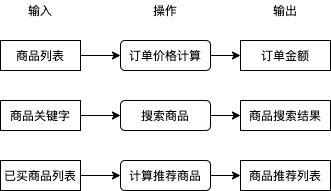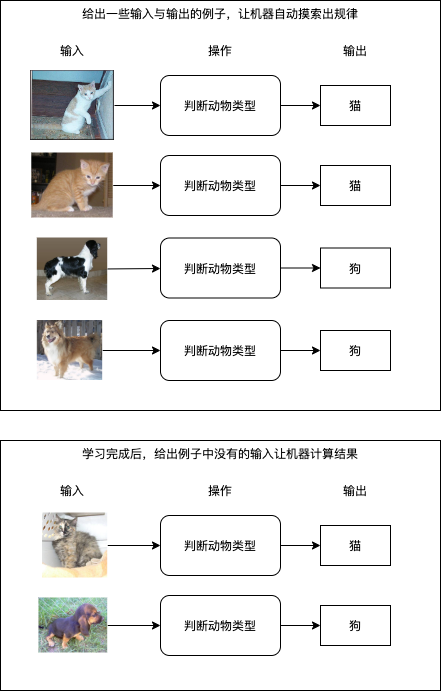• 做不到 100% 的精度，例如前述的根据商品列表计算订单价格要求非常准确，我们不能用机器学习来实现这个操作
• 需要一定的数据量，如果例子较少则无法成功学习到规律
• 无法实现复杂的判断，机器学习与人脑之间仍然有相当大的差距，一些复杂的操作无法使用机器学习代替

## 机器学习的流程与步骤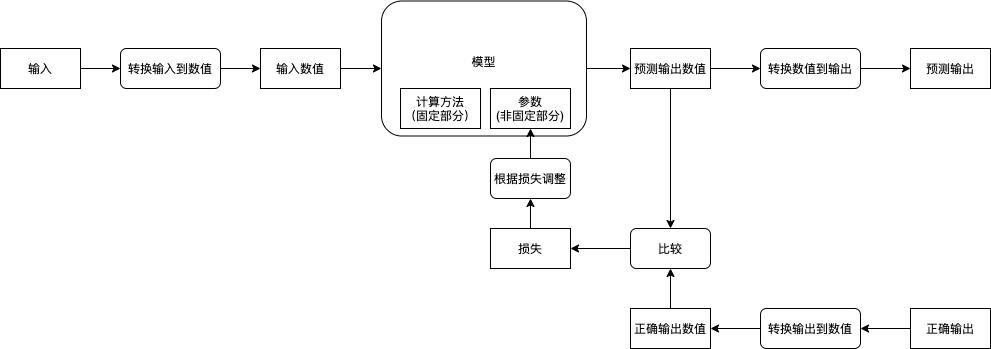• 收集输入与输出的例子
• 建立模型
• 确定输入输出与模型可接收返回的数值之间如何转换
• 使用输入与输出的例子调整模型的参数
• 使用没有参与训练的输入与输出评价模型是否成功摸索出规律

### 收集输入与输出的例子

https://github.com/awesomedata/awesome-public-datasets

### 使用输入与输出的例子调整模型的参数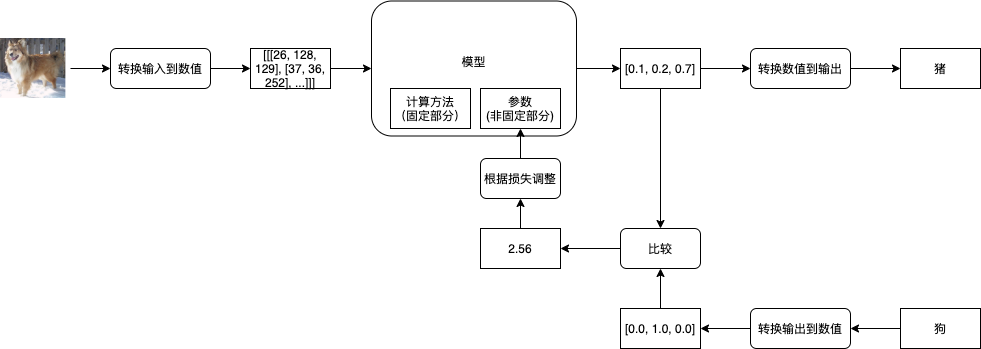### 使用没有参与训练的输入与输出评价模型是否成功摸索出规律

• 第一轮训练后，训练集正确率 60 %，验证集正确率 58 %
• 第二轮训练后，训练集正确率 79 %，验证集正确率 72 %
• 第三轮训练后，训练集正确率 88 %，验证集正确率 86 %
• 第四轮训练后，训练集正确率 92 %，验证集正确率 85 %
• 第五轮训练后，训练集正确率 99 %，验证集正确率 78 %

• 训练集 (Training Set): 用于训练模型参数
• 验证集 (Validating Set): 用于判断模型是否支持处理没有训练过的输入，并手动调整模型的计算方法
• 测试集 (Testing Set): 用于最终判断模型是否支持处理完全没有参与训练与手动调整模型的输入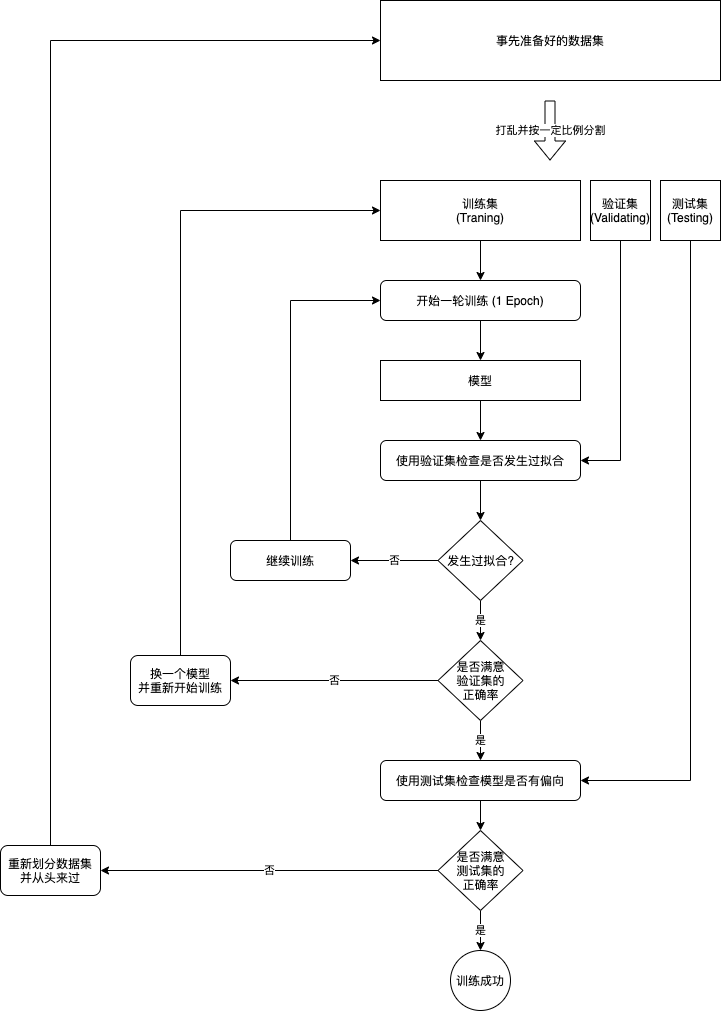## 一个最简单的例子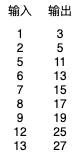• 用 x 代表输入
• 用 y 代表输出
• 用 weight 代表输入乘以的值 (公式中缩写为 w)
• 用 bias 代表输出加上的值 (公式中缩写为 b)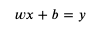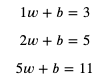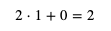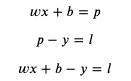• weight 增加 1 时，loss 会增加 x
• bias 增加 1 时，loss 会增加 1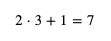• weight 增加 x 乘以 0.01
• bias 增加 0.01

• weight 减少 x 乘以 0.01
• bias 减少 0.01

第一轮：
x = 2, y = 5, weight = 1, bias = 0
predicted = 2 * 1 + 0 = 2
loss = 2 - 5 = -3
weight += 2 * 0.1
bias += 0.1

x = 2, y = 5, weight = 1.02, bias = 0.01
predicted = 2 * 1.02 + 0.01 = 2.05
loss = 2.05 - 5 = -2.95
weight += 2 * 0.1
bias += 0.1

x = 2, y = 5, weight = 1.04, bias = 0.02
predicted = 2 * 1.04 + 0.02 = 2.1
loss = 2.1 - 5 = -2.9
weight += 2 * 0.1
bias += 0.1


# 定义参数
weight = 1
bias = 0

# 定义学习比率
learning_rate = 0.01

# 准备训练集，验证集和测试集
traning_set = [(2, 5), (5, 11), (6, 13), (7, 15), (8, 17)]
validating_set = [(12, 25), (1, 3)]
testing_set = [(9, 19), (13, 27)]

for epoch in range(1, 10000):
print(f"epoch: {epoch}")

# 根据训练集训练并修改参数
for x, y in traning_set:
# 计算预测值
predicted = x * weight + bias
# 计算损失
loss = predicted - y
# 打印除错信息
print(f"traning x: {x}, y: {y}, predicted: {predicted}, loss: {loss}, weight: {weight}, bias: {bias}")
# 判断需要如何修改 weight 和 bias 才能减少 loss
if loss < 0:
# 需要增加 weight 和 bias 来让 predicted 更大
weight += x * learning_rate
bias += 1 * learning_rate
else:
# 需要减少 weight 和 bias 来让 predicted 更小
weight -= x * learning_rate
bias -= 1 * learning_rate

# 检查验证集
validating_accuracy = 0
for x, y in validating_set:
predicted = x * weight + bias
validating_accuracy += 1 - abs(y - predicted) / y
print(f"validating x: {x}, y: {y}, predicted: {predicted}")
validating_accuracy /= len(validating_set)

# 如果验证集正确率大于 99 %，则停止训练
print(f"validating accuracy: {validating_accuracy}")
if validating_accuracy > 0.99:
break

# 检查测试集
testing_accuracy = 0
for x, y in testing_set:
predicted = x * weight + bias
testing_accuracy += 1 - abs(y - predicted) / y
print(f"testing x: {x}, y: {y}, predicted: {predicted}")
testing_accuracy /= len(testing_set)
print(f"testing accuracy: {testing_accuracy}")


epoch: 1
traning x: 2, y: 5, predicted: 2, loss: -3, weight: 1, bias: 0
traning x: 5, y: 11, predicted: 5.109999999999999, loss: -5.890000000000001, weight: 1.02, bias: 0.01
traning x: 6, y: 13, predicted: 6.4399999999999995, loss: -6.5600000000000005, weight: 1.07, bias: 0.02
traning x: 7, y: 15, predicted: 7.940000000000001, loss: -7.059999999999999, weight: 1.1300000000000001, bias: 0.03
traning x: 8, y: 17, predicted: 9.64, loss: -7.359999999999999, weight: 1.2000000000000002, bias: 0.04
validating x: 12, y: 25, predicted: 15.410000000000004
validating x: 1, y: 3, predicted: 1.3300000000000003
validating accuracy: 0.5298666666666668
epoch: 2
traning x: 2, y: 5, predicted: 2.6100000000000003, loss: -2.3899999999999997, weight: 1.2800000000000002, bias: 0.05
traning x: 5, y: 11, predicted: 6.560000000000001, loss: -4.439999999999999, weight: 1.3000000000000003, bias: 0.060000000000000005
traning x: 6, y: 13, predicted: 8.170000000000002, loss: -4.829999999999998, weight: 1.3500000000000003, bias: 0.07
traning x: 7, y: 15, predicted: 9.950000000000003, loss: -5.049999999999997, weight: 1.4100000000000004, bias: 0.08
traning x: 8, y: 17, predicted: 11.930000000000003, loss: -5.069999999999997, weight: 1.4800000000000004, bias: 0.09
validating x: 12, y: 25, predicted: 18.820000000000007
validating x: 1, y: 3, predicted: 1.6600000000000006
validating accuracy: 0.6530666666666669
epoch: 3
traning x: 2, y: 5, predicted: 3.220000000000001, loss: -1.779999999999999, weight: 1.5600000000000005, bias: 0.09999999999999999
traning x: 5, y: 11, predicted: 8.010000000000002, loss: -2.9899999999999984, weight: 1.5800000000000005, bias: 0.10999999999999999
traning x: 6, y: 13, predicted: 9.900000000000002, loss: -3.099999999999998, weight: 1.6300000000000006, bias: 0.11999999999999998
traning x: 7, y: 15, predicted: 11.960000000000004, loss: -3.0399999999999956, weight: 1.6900000000000006, bias: 0.12999999999999998
traning x: 8, y: 17, predicted: 14.220000000000006, loss: -2.779999999999994, weight: 1.7600000000000007, bias: 0.13999999999999999
validating x: 12, y: 25, predicted: 22.230000000000008
validating x: 1, y: 3, predicted: 1.9900000000000007
validating accuracy: 0.7762666666666669

epoch: 90
traning x: 2, y: 5, predicted: 4.949999999999935, loss: -0.05000000000006466, weight: 1.9799999999999676, bias: 0.9900000000000007
traning x: 5, y: 11, predicted: 10.999999999999838, loss: -1.616484723854228e-13, weight: 1.9999999999999676, bias: 1.0000000000000007
traning x: 6, y: 13, predicted: 13.309999999999807, loss: 0.3099999999998069, weight: 2.0499999999999674, bias: 1.0100000000000007
traning x: 7, y: 15, predicted: 14.929999999999772, loss: -0.07000000000022766, weight: 1.9899999999999674, bias: 1.0000000000000007
traning x: 8, y: 17, predicted: 17.48999999999974, loss: 0.4899999999997391, weight: 2.059999999999967, bias: 1.0100000000000007
validating x: 12, y: 25, predicted: 24.759999999999607
validating x: 1, y: 3, predicted: 2.9799999999999676
validating accuracy: 0.9918666666666534
testing x: 9, y: 19, predicted: 18.819999999999705
testing x: 13, y: 27, predicted: 26.739999999999572
testing accuracy: 0.9904483430799063


pip3 install matplotlib


# 定义参数
weight = 1
bias = 0

# 定义学习比率
learning_rate = 0.01

# 准备训练集，验证集和测试集
traning_set = [(2, 5), (5, 11), (6, 13), (7, 15), (8, 17)]
validating_set = [(12, 25), (1, 3)]
testing_set = [(9, 19), (13, 27)]

# 记录 weight 与 bias 的历史值
weight_history = [weight]
bias_history = [bias]

for epoch in range(1, 10000):
print(f"epoch: {epoch}")

# 根据训练集训练并修改参数
for x, y in traning_set:
# 计算预测值
predicted = x * weight + bias
# 计算损失
loss = predicted - y
# 打印除错信息
print(f"traning x: {x}, y: {y}, predicted: {predicted}, loss: {loss}, weight: {weight}, bias: {bias}")
# 判断需要如何修改 weight 和 bias 才能减少 loss
if loss < 0:
# 需要增加 weight 和 bias 来让 predicted 更大
weight += x * learning_rate
bias += 1 * learning_rate
else:
# 需要减少 weight 和 bias 来让 predicted 更小
weight -= x * learning_rate
bias -= 1 * learning_rate
weight_history.append(weight)
bias_history.append(bias)

# 检查验证集
validating_accuracy = 0
for x, y in validating_set:
predicted = x * weight + bias
validating_accuracy += 1 - abs(y - predicted) / y
print(f"validating x: {x}, y: {y}, predicted: {predicted}")
validating_accuracy /= len(validating_set)

# 如果验证集正确率大于 99 %，则停止训练
print(f"validating accuracy: {validating_accuracy}")
if validating_accuracy > 0.99:
break

# 检查测试集
testing_accuracy = 0
for x, y in testing_set:
predicted = x * weight + bias
testing_accuracy += 1 - abs(y - predicted) / y
print(f"testing x: {x}, y: {y}, predicted: {predicted}")
testing_accuracy /= len(testing_set)
print(f"testing accuracy: {testing_accuracy}")

# 显示 weight 与 bias 的变化
from matplotlib import pyplot
pyplot.plot(weight_history, label="weight")
pyplot.plot(bias_history, label="bias")
pyplot.legend()
pyplot.show()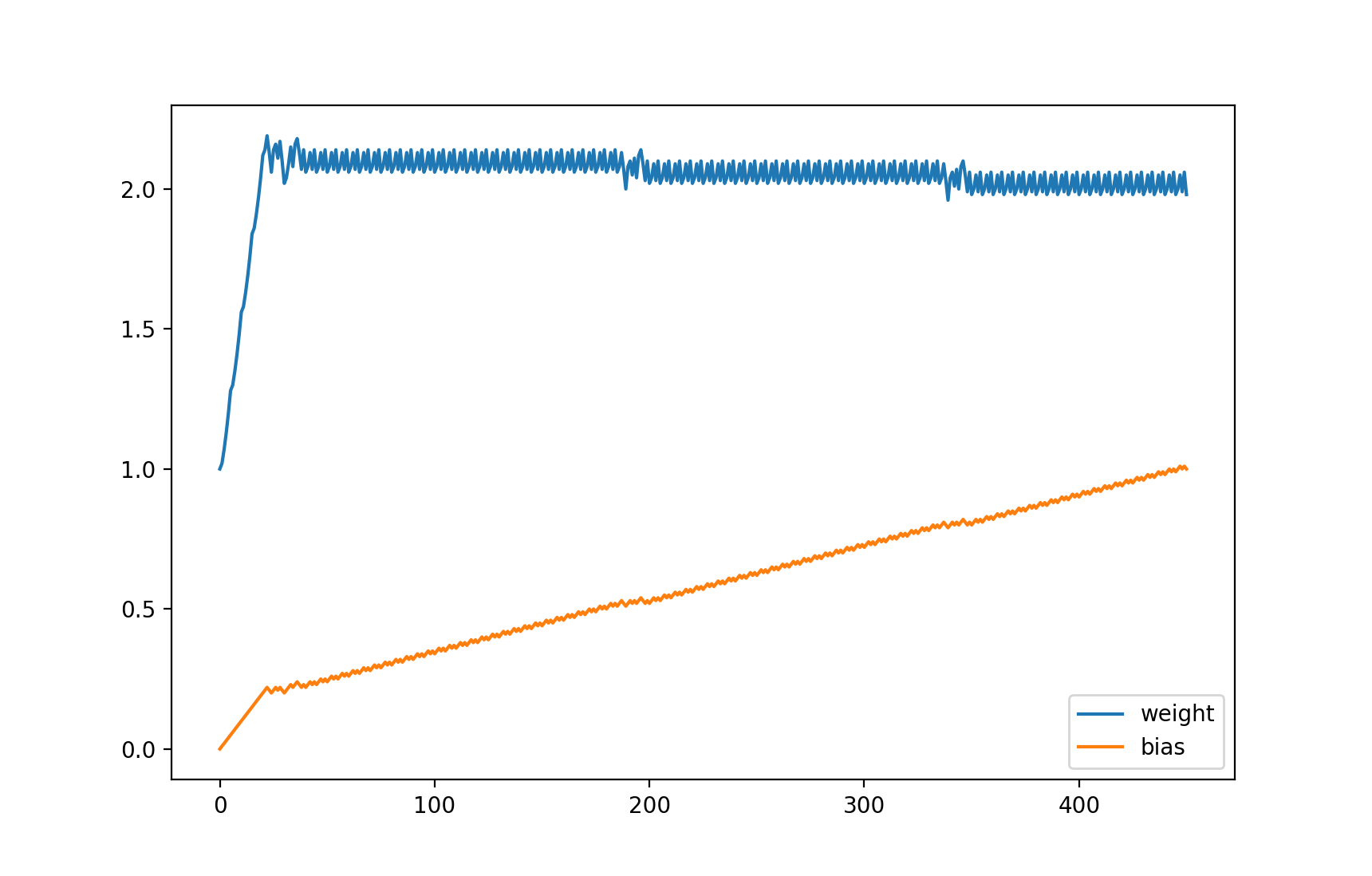## 机器学习与微分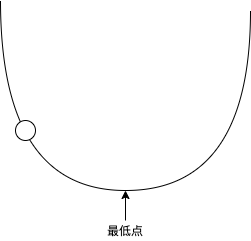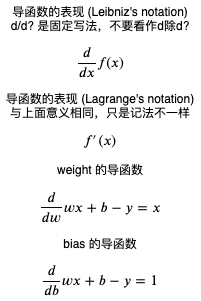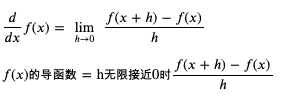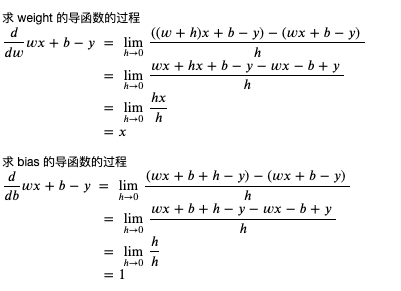当 x 等于 3 时，x 的平方等于 9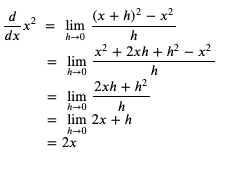>>> ((3 + 1) ** 2 - 3 ** 2) / 1
7.0
>>> ((3 + 0.1) ** 2 - 3 ** 2) / 0.1
6.100000000000012
>>> ((3 + 0.01) ** 2 - 3 ** 2) / 0.01
6.009999999999849
>>> ((3 + 0.001) ** 2 - 3 ** 2) / 0.001
6.000999999999479
>>> ((5 + 1) ** 2 - 5 ** 2) / 1
11.0
>>> ((5 + 0.1) ** 2 - 5 ** 2) / 0.1
10.09999999999998
>>> ((5 + 0.01) ** 2 - 5 ** 2) / 0.01
10.009999999999764
>>> ((5 + 0.001) ** 2 - 5 ** 2) / 0.001
10.001000000002591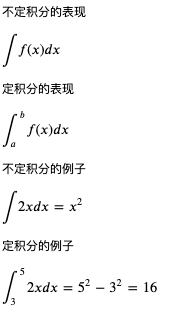• 假设一个可以从输入计算输出的公式
• 定好计算损失的方法，并把公式变形为计算损失的公式
• 利用微分来计算公式的各个参数对损失的贡献比例 (也就是偏导)
• 随机分配参数的值
• 用预先收集好的输入计算预测输出，然后用预测输出和正确输出计算损失
• 根据各个参数对损失的贡献比例调整参数，使得损失接近 0
• 损失非常接近 0 时，代表公式计算的预测输出非常接近正确输出，如果达到可接受的范围就可以停止训练

## 让参数调整量依赖损失的大小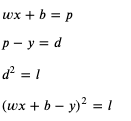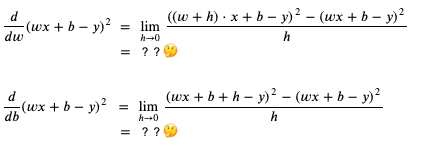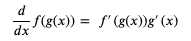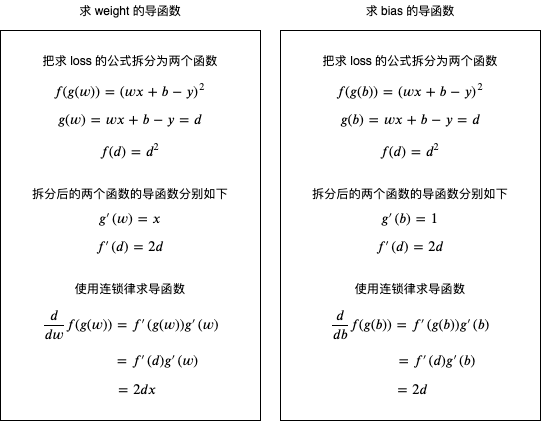# 定义参数
weight = 1
bias = 0

# 定义学习比率
learning_rate = 0.01

# 准备训练集，验证集和测试集
traning_set = [(2, 5), (5, 11), (6, 13), (7, 15), (8, 17)]
validating_set = [(12, 25), (1, 3)]
testing_set = [(9, 19), (13, 27)]

# 记录 weight 与 bias 的历史值
weight_history = [weight]
bias_history = [bias]

for epoch in range(1, 10000):
print(f"epoch: {epoch}")

# 根据训练集训练并修改参数
for x, y in traning_set:
# 计算预测值
predicted = x * weight + bias
# 计算损失
diff = predicted - y
loss = diff ** 2
# 打印除错信息
print(f"traning x: {x}, y: {y}, predicted: {predicted}, loss: {loss}, weight: {weight}, bias: {bias}")
# 计算导函数值
derivative_weight = 2 * diff * x
derivative_bias = 2 * diff
# 修改 weight 和 bias 以减少 loss
# diff 为正时代表预测输出 > 正确输出，会减少 weight 和 bias
# diff 为负时代表预测输出 < 正确输出，会增加 weight 和 bias
weight -= derivative_weight * learning_rate
bias -= derivative_bias * learning_rate
# 记录 weight 和 bias 的历史值
weight_history.append(weight)
bias_history.append(bias)

# 检查验证集
validating_accuracy = 0
for x, y in validating_set:
predicted = x * weight + bias
validating_accuracy += 1 - abs(y - predicted) / y
print(f"validating x: {x}, y: {y}, predicted: {predicted}")
validating_accuracy /= len(validating_set)

# 如果验证集正确率大于 99 %，则停止训练
print(f"validating accuracy: {validating_accuracy}")
if validating_accuracy > 0.99:
break

# 检查测试集
testing_accuracy = 0
for x, y in testing_set:
predicted = x * weight + bias
testing_accuracy += 1 - abs(y - predicted) / y
print(f"testing x: {x}, y: {y}, predicted: {predicted}")
testing_accuracy /= len(testing_set)
print(f"testing accuracy: {testing_accuracy}")

# 显示 weight 与 bias 的变化
from matplotlib import pyplot
pyplot.plot(weight_history, label="weight")
pyplot.plot(bias_history, label="bias")
pyplot.legend()
pyplot.show()


epoch: 1
traning x: 2, y: 5, predicted: 2, loss: 9, weight: 1, bias: 0
traning x: 5, y: 11, predicted: 5.66, loss: 28.5156, weight: 1.12, bias: 0.06
traning x: 6, y: 13, predicted: 10.090800000000002, loss: 8.463444639999992, weight: 1.6540000000000001, bias: 0.1668
traning x: 7, y: 15, predicted: 14.246711999999999, loss: 0.567442810944002, weight: 2.003104, bias: 0.22498399999999996
traning x: 8, y: 17, predicted: 17.108564320000003, loss: 0.011786211577063013, weight: 2.10856432, bias: 0.24004976
validating x: 12, y: 25, predicted: 25.332206819199993
validating x: 1, y: 3, predicted: 2.3290725023999994
validating accuracy: 0.8815346140160001
epoch: 2
traning x: 2, y: 5, predicted: 4.420266531199999, loss: 0.3360908948468813, weight: 2.0911940287999995, bias: 0.23787847359999995
traning x: 5, y: 11, predicted: 10.821389980735997, loss: 0.03190153898148744, weight: 2.1143833675519996, bias: 0.24947314297599996
traning x: 6, y: 13, predicted: 13.046511560231679, loss: 0.0021633252351850635, weight: 2.1322443694784, bias: 0.25304534336128004
traning x: 7, y: 15, predicted: 15.138755987910837, loss: 0.019253224181112433, weight: 2.1266629822505987, bias: 0.25211511215664645
traning x: 8, y: 17, predicted: 17.10723714394308, loss: 0.011499805041069082, weight: 2.1072371439430815, bias: 0.2493399923984297
validating x: 12, y: 25, predicted: 25.32814566046583
validating x: 1, y: 3, predicted: 2.3372744504317566
validating accuracy: 0.8829828285293095
epoch: 3
traning x: 2, y: 5, predicted: 4.427353651343945, loss: 0.327923840629112, weight: 2.0900792009121885, bias: 0.24719524951956806
traning x: 5, y: 11, predicted: 10.823573450784844, loss: 0.03112632726796794, weight: 2.112985054858431, bias: 0.2586481764926892
traning x: 6, y: 13, predicted: 13.045942966156671, loss: 0.0021107561392730407, weight: 2.1306277097799464, bias: 0.2621767074769923
traning x: 7, y: 15, predicted: 15.13705972504188, loss: 0.01878536822855566, weight: 2.125114553841146, bias: 0.2612578481538589
traning x: 8, y: 17, predicted: 17.105926192335282, loss: 0.011220358222651178, weight: 2.1059261923352826, bias: 0.2585166536530213
validating x: 12, y: 25, predicted: 25.324134148545966
validating x: 1, y: 3, predicted: 2.3453761313679533
validating accuracy: 0.8844133389237396

epoch: 202
traning x: 2, y: 5, predicted: 4.950471765167672, loss: 0.002453046045606255, weight: 2.0077909582882314, bias: 0.9348898485912089
traning x: 5, y: 11, predicted: 10.984740851695477, loss: 0.00023284160697942092, weight: 2.0097720876815246, bias: 0.9358804132878555
traning x: 6, y: 13, predicted: 13.003973611325808, loss: 1.578958696858945e-05, weight: 2.011298002511977, bias: 0.936185596253946
traning x: 7, y: 15, predicted: 15.011854308097591, loss: 0.00014052462047262272, weight: 2.01082116915288, bias: 0.9361061240274299
traning x: 8, y: 17, predicted: 17.009161566019216, loss: 8.393429192445584e-05, weight: 2.0091615660192175, bias: 0.935869037865478
validating x: 12, y: 25, predicted: 25.02803439201881
validating x: 1, y: 3, predicted: 2.9433815220012365
validating accuracy: 0.9900028991598299
testing x: 9, y: 19, predicted: 19.00494724565038
testing x: 13, y: 27, predicted: 27.03573010747495
testing accuracy: 0.9992081406680464


weight 与 bias 的变化如下: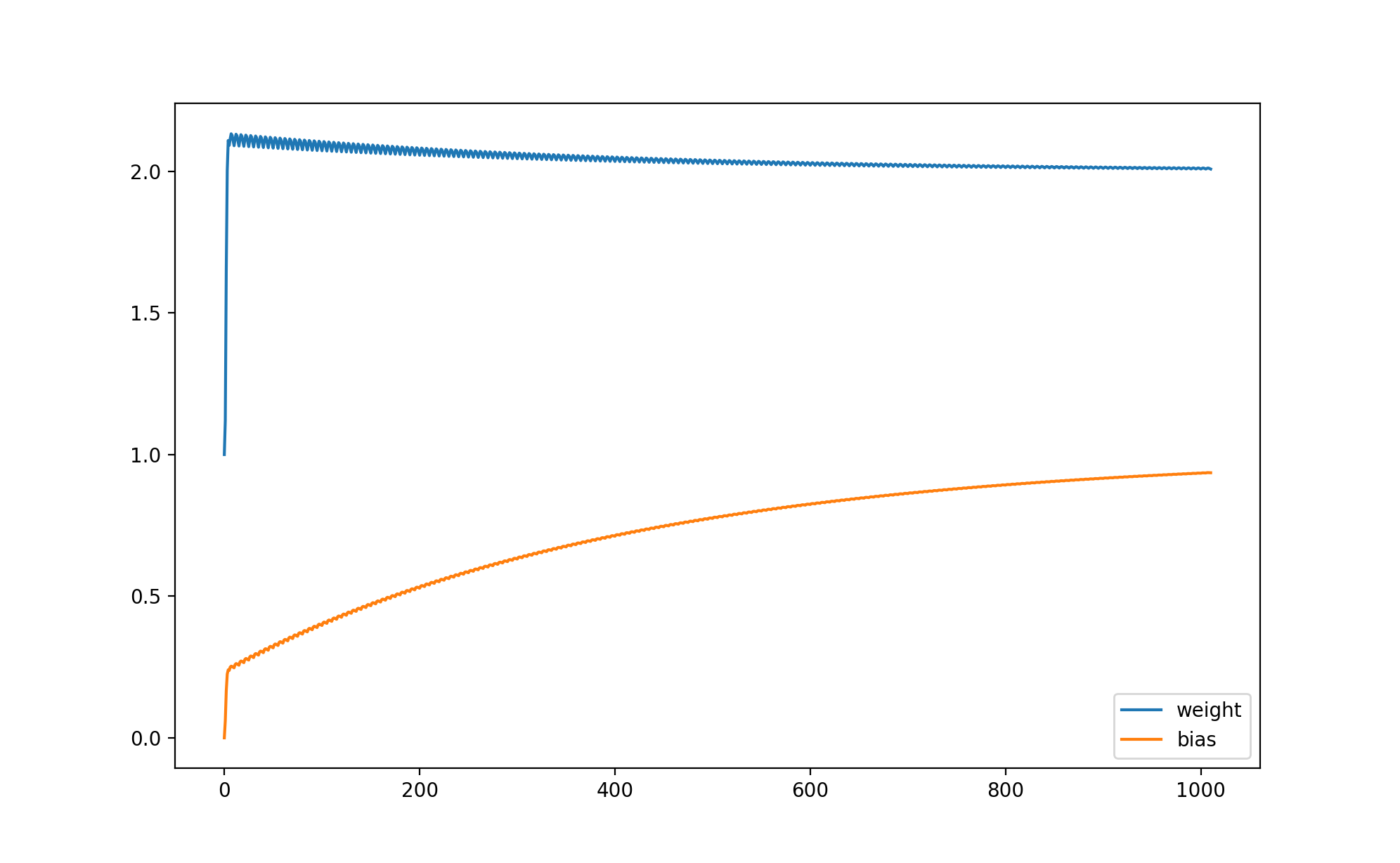## 写在最后

• pytorch 与矩阵计算入门
• 线性模型，激活函数与多层线性模型
• 递归神经网络 RNN, LSTM 与 GRU
• 卷积神经网络 CNN
• (之后如果还有时间可能会介绍 R-CNN, Transformer, GAN 等更复杂的模型)

posted on 2020-04-06 16:35  q303248153  阅读(3349)  评论(13编辑  收藏